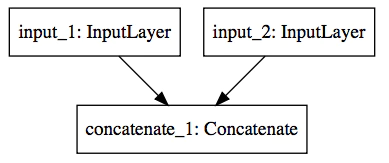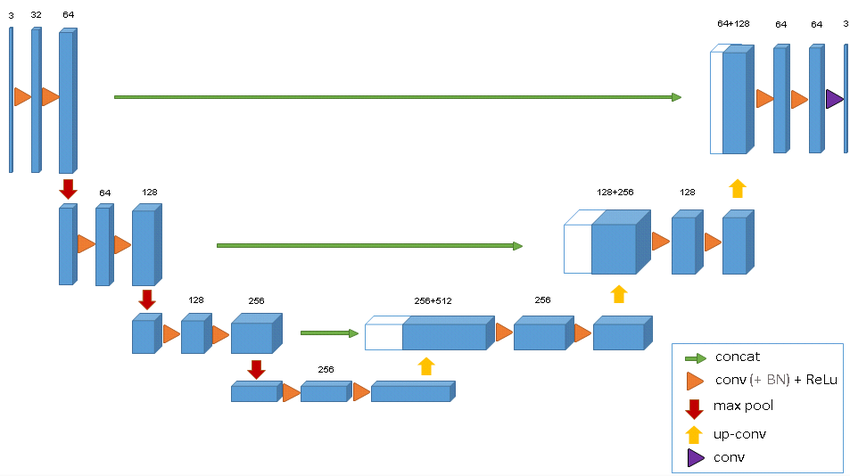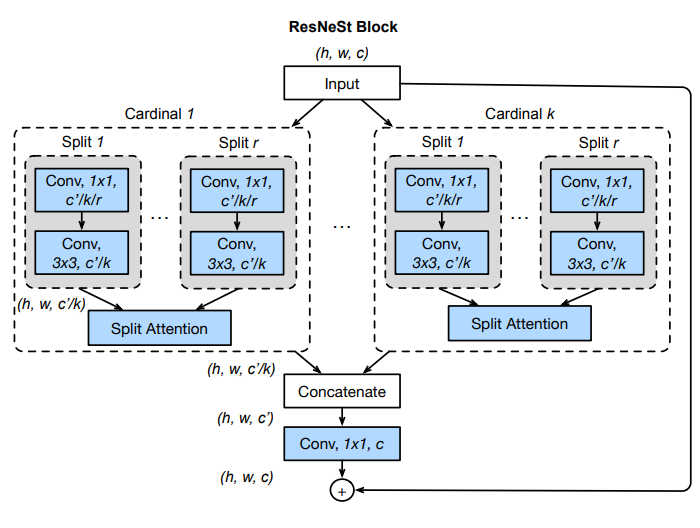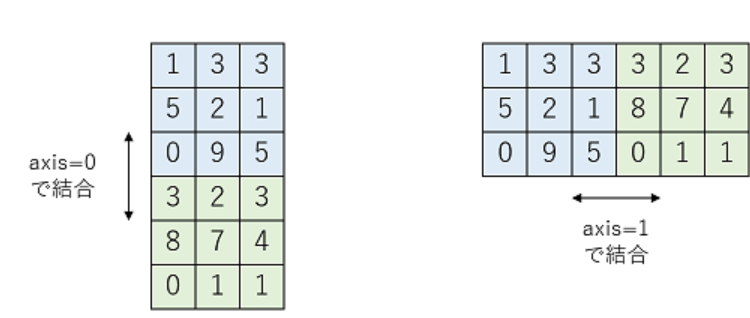# Introduction

If you read Keras's F. Chollet book, there is a part that explains Concatenate Layer. It is represented in the picture below, but it was a refreshing idea of what kind of calculation was performed in this.By the way, since Concatenate Layer plays an important role in U-NET, Resnet, etc., it is not good to leave it without understanding Concatenate Layer any more, so use a simple tensor and use Concatenate Layer. I decided to check the behavior.Figure U-Net ArchitectureFigure ResNet block

# Understanding

In Excel, I used the Concatenate function at the time of string concatenation, so I had an image of ** joining multiple arrays without any operation **, but [Teratail](https: /) /teratail.com/questions/163385) has an easy-to-understand diagram, so I will introduce it here.

Here, there are two matrices, blue and green. These matrices are two-dimensional (2D) tensors, and the Shapes of these matrices are (3,3). axis refers to the dimension of the tensor. [Understanding Tensor (2): Shape]![Image.png](https://qiita-image-store.s3.ap-northeast-1.amazonaws.com/0/208980/9c722160-613a- 1df8-6773-ad133d433db8.png)

axis also refers to the dimensional axis of the tensor. (It may be good to think of the vector when expressing the moment in physics.)

In the case of a two-dimensional tensor, axis = 0 means the vertical direction and axis = 1 means the horizontal direction.

However, when axis = -1, it means the last axis. Consider a slice of a Python list.

Then, at the time of Concatenate Layer, it is possible to specify the connection direction.

** (1) When axis = 0, join vertically. ** **

** (2) If axis = 1, join horizontally. (However, in the case of 2D, axis = -1 has the same meaning) **# Program code

#### `python`

``````

import tensorflow as tf
import numpy as np

#Preparing for 2D Tensor
x1 = np.array([[1,3,3],
[5,2,1],
[0,9,5]])

x2 = np.array([[3,2,3],
[8,7,4],
[0,1,1]])

print('x1=',x1)
print('x2=',x2)

``````

Concatenate Layer

### Vertical connection

``````#Concantenate Layer
# axis = 0,Vertical coupling
y1 = tf.keras.layers.Concatenate(axis=0)([x1,x2])
print('y1=',y1)
``````

#### `Vertical join result`

``````
y1= tf.Tensor(
[[1 3 3]
[5 2 1]
[0 9 5]
[3 2 3]
[8 7 4]
[0 1 1]], shape=(6, 3), dtype=int32)

``````

### Horizontal connection

``````# axis = 1 (or axis = -1)Horizontal coupling
y2 = tf.keras.layers.Concatenate(axis=-1)([x1,x2])
print('y2=',y2)
``````

#### `Horizontal connection result`

``````
y2= tf.Tensor(
[[1 3 3 3 2 3]
[5 2 1 8 7 4]
[0 9 5 0 1 1]], shape=(3, 6), dtype=int32)

``````

# Summary

I finally understood the operation of Concatenate Layer. (I feel.) However, Concatenate's spelling is hard to come by. (Tears)